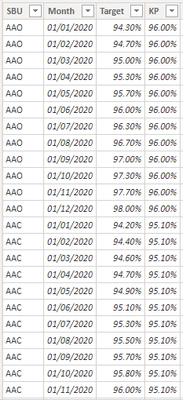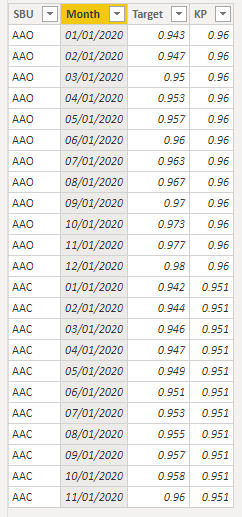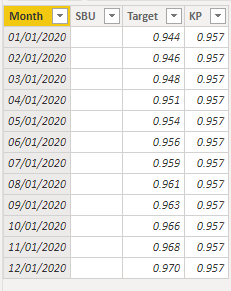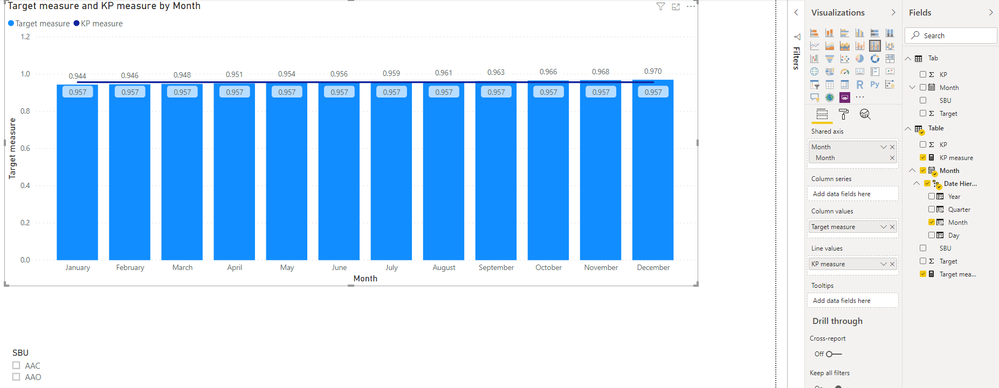cancel
Showing results for
Did you mean:Anonymous
Not applicable

## KPI calculated measure

I asked this question before but i didn't explained it properly.
so i have a table( "TABLE 1") having 4 columns, i wanted to created an kpi using Line and clustered column chart.

the table contains target for different SBU for different months of the year 2020.I have created another table with same column names,but the SBU is empty along with target.(TABLE 2)So In my line and clustered column chart when no SBU is selected it should show values from Table 2 and when SBU is selected it should show the Maximum value of that particular SBU for all the months.but i don't know how to create a measure for this particular problem.In the above visual when No SBU is selected it is supposed to show 94.40 % for the month of january from TABLE 2 ,Instead it's showing 94.20% because it's taking the average of all SBU for the month january of TABLE 1

3 REPLIES 3Community Support

Hi, @Anonymous

If you take the answer of someone, please mark it as the solution to help the other members who have same problems find it more quickly. If not, let me know and I'll try to help you further. Thanks.

Best Regards

AllanCommunity Support

Hi, @Anonymous

Tab:Table:You may create two measures as below.

``````Target measure =
var _month = SELECTEDVALUE('Table'[Month].[Month])
var _sbu = SELECTEDVALUE(Tab[SBU])
return
IF(
NOT(ISFILTERED(Tab[SBU])),
CALCULATE(
SUM('Table'[Target]),
FILTER(
ALLSELECTED('Table'),
'Table'[Month].[Month] = _month
)
),
IF(
HASONEVALUE(Tab[SBU]),
CALCULATE(
MAX(Tab[Target]),
FILTER(
ALLSELECTED(Tab),
Tab[SBU] = _sbu&&
Tab[Month].[Month] = _month
)
)
)
)

KP measure =
var _month = SELECTEDVALUE('Table'[Month].[Month])
var _sbu = SELECTEDVALUE(Tab[SBU])
return
IF(
NOT(ISFILTERED(Tab[SBU])),
CALCULATE(
SUM('Table'[KP]),
FILTER(
ALLSELECTED('Table'),
'Table'[Month].[Month] = _month
)
),
IF(
HASONEVALUE(Tab[SBU]),
CALCULATE(
MAX(Tab[KP]),
FILTER(
ALLSELECTED(Tab),
Tab[SBU] = _sbu&&
Tab[Month].[Month] = _month
)
)
)
)``````

Result:Best Regards

Allan

If this post helps, then please consider Accept it as the solution to help the other members find it more quickly.Super User